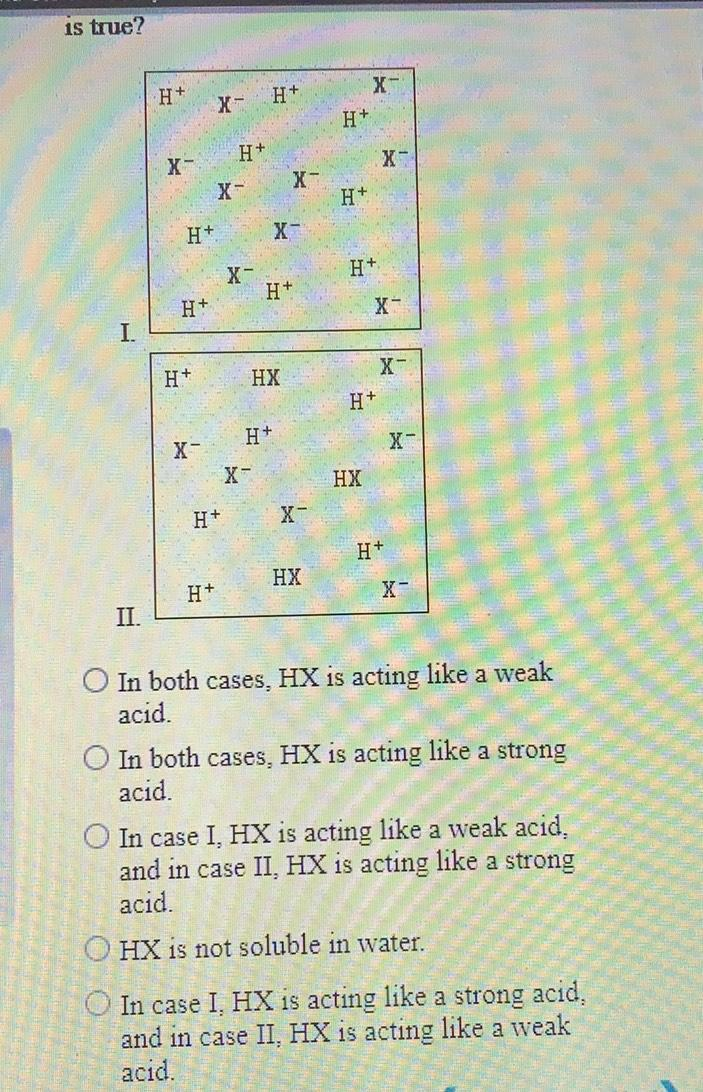Question:

# is true? H+ - H+ H+ H+ X- X- X- H+ H+ X- X- H+ H+ H+ I. X- H+ HX H+ H+ <- X x HX H+ X- H+ HX H+ X II. In both cases. HX is actinis true? H+ - H+ H+ H+ X- X- X- H+ H+ X- X- H+ H+ H+ I. X- H+ HX H+ H+ <- X x HX H+ X- H+ HX H+ X II. In both cases. HX is acting like a weak acid. O In both cases, HX is acting like a strong acid. In case I, HX is acting like a weak acid. and in case II, HX is acting like a strong acid. O HX is not soluble in water. In case I. HX is acting like a strong acid. and in case II, HX is acting like a weak acid.